##### Differential Equations For DummiesWhen you use integration to calculate arc length, what you’re doing (sort of) is dividing a length of curve into infinitesimally small sections, figuring the length of each small section, and then adding up all the little lengths. The following figure shows how each section of a curve can be approximated by the hypotenuse of a tiny right triangle.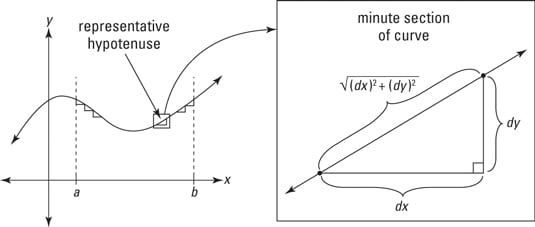The Pythagorean Theorem is the key to the arc length formula.

You can imagine that as you zoom in further and further on a curve, dividing the curve into more and more sections, the minute sections get straighter and straighter and the hypotenuses of the right triangles better and better approximate the curve. That’s why — when this process of adding up smaller and smaller sections is taken to the limit — you get the precise length of the curve.

So, all you have to do is add up all the hypotenuses along the curve between your start and finish points. The lengths of the legs of each infinitesimal triangle are dx and dy, and thus the length of the hypotenuse — given by the Pythagorean Theorem — is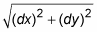To add up all the hypotenuses from a to b along the curve, you just integrate: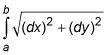But first, you’ve got to tweak this a bit to produce the formula for arc length.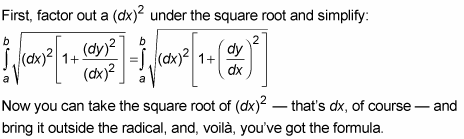The arc length along a curve, y = f(x), from a to b, is given by the following integral: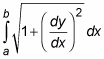The expression inside this integral is simply the length of a representative hypotenuse.

Try this one: What’s the length along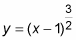from x = 1 to x = 5?

1. Take the derivative of your function.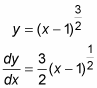2. Plug this into the formula and integrate.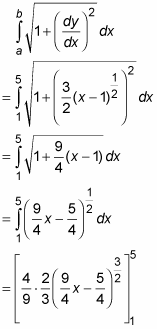(See how this works? It’s the guess-and-check integration technique with the reverse power rule. The 4/9 is the tweak amount you need because of the coefficient 9/4.)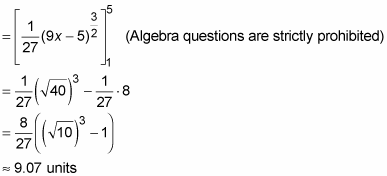Now, if you ever find yourself on a road with the shape of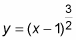and your odometer’s broken, you can figure the exact length of your drive. Your friends will be very impressed — or very concerned.